Monthly Tech-Tip from Tony Hansen SignUp No tracking! No ads!

# How desktop INSIGHT Deals With Unity, LOI and Formula Weight

#### Description

INSIGHT enables you to enter material analyses as recipes. This is a first step to inserting them into the materials database. Imposing an LOI and understanding how to set unity, and its connection with formula weight are important concepts.

#### Article

Sometimes the relationship between the way INSIGHT calculates the formula of certain materials and the way it stores them in the materials table can be puzzling. This is especially true with clays. It is important to understand this because the best way to enter materials into INSIGHTs materials database is by first entering their chemistry as a recipe.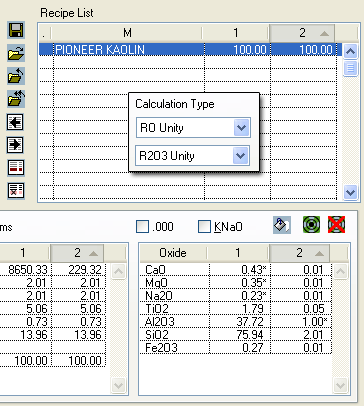Figure 1

Consider the example of Pioneer Kaolin, a material already known by INSIGHT. This screen image shows a one-material-recipe duplicated into recipe 2 calculated as RO Unity on the left and R2O3 unity on the right. Even though these numbers look so different the chemistry for the two recipes is exactly the same, it is the unity is that is different.

Setting RO unity is not really appropriate for kaolins because recalculating the minute amount of fluxes to unity drives the alumina and silica way up and thus the formula weight is also very high. In addition, if you make even a small change to one of the flux amounts the silica and alumina numbers will change drastically. It is thus better to set the alumina at unity, then the weight will be in the expected range. If you consider that theoretical kaolin has no flux, this makes sense.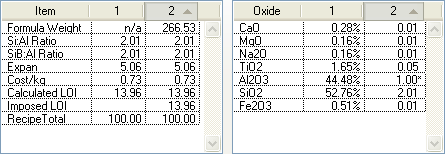Figure 2

However, remember that INSIGHT remembers materials as formulas in its materials database and calculations will still work properly no matter what unity setting a material is recorded with. This is true because the proportion of oxides one to another does not change with unity setting changes.

Notice that INSIGHT shows LOI as 13.96 for both recipes on the last line of the Property list (lower left figure 1). INSIGHT knows the LOI of each material in its materials table by noting the difference between the formula weight it calculates and the one it has been given to save with the material (it assumes any difference is LOI). The 13.96 is the calculated LOI of the mix of materials in the unfired recipe. However INSIGHT also shows LOI as zero above the Property list (center left). Why? This is the LOI that we can impose on INSIGHT (forcing it to LOI adjust the formula weight). INSIGHT normally calculates formula weight using a zero LOI because it is assuming you want to know the chemistry of the fired product (which has no LOI).

In figure 2 I have set recipe 1 to display analysis and used the "Override Calculated LOI" item in the Calc menu to impose at LOI of 13.96 on recipe 2 only. Notice the formula weight for recipe 2 has increased to a typical kaolin value.* That means that raw unfired kaolin is now properly represented in recipe 2 (and ready to be inserted into the materials table if desired). Notice that recipe 1 shows no formula weight. This is because an analysis cannot, by definition, have a weight.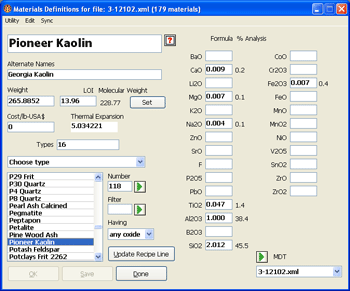Figure 3

Figure 3 shows the material record for the same kaolin. The formula and percentage analysis numbers are shown for each oxide. You can compare these and the weight, molecular weight and LOI numbers to confirm some of the things just discussed. However why are the percentages in the materials dialog different that those in recipe 1 in Figure 2? Because I did not impose an LOI on recipe 1, if I would have they would be the same.

Do you need to know all this stuff? Not really, INSIGHT will do its calculations correctly as long as it is given an LOI compensated weight for materials entered into the materials table. There is an example in the INSIGHT manual of adding a material that shows you how to do this most effectively.

One final point: Why are there differences in second decimal in some of the numbers shown here? The answer: INSIGHT favors calculation speed over unneeded accuracy.

## Related Information

### Imposing an LOI in INSIGHT

Tap picture for full size and resolution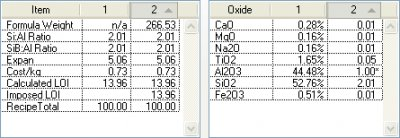Desktop Insight can calculate the LOI of a recipe based on the LOIs it knows of the individual materials in the recipe. But sometimes you need to impose an LOI to force a calculated analysis to match an actual measured LOI in the lab.

### Desktop INSIGHT MDT dialog showing kaolin LOI

Tap picture for full size and resolution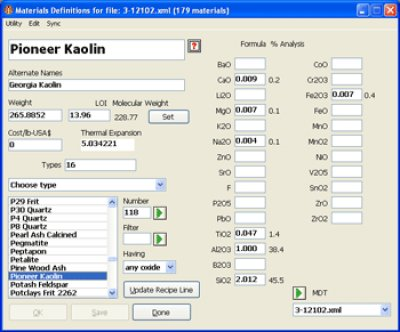The LOI appears below the material name and alternative names (beside the weight). The formula that goes with that LOI is the bold numbers in the blanks beside the oxide names on the right.

 By Tony Hansen